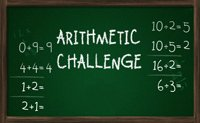Arithmetic Challenge

 Arithmetic ChallengeIn Arithmetic Challenge we can choose one of four operators, so - / and got 1 minute to solve the tasks. It's a little math game with addition, subtraction, division and divide.

Tags:
puzzle